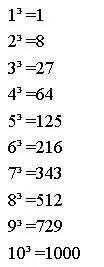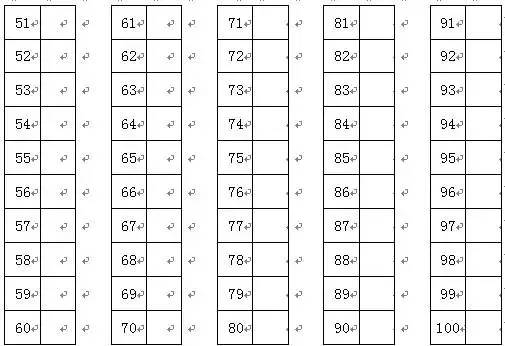# [试题分享] 六个数学技巧,帮助孩子提高计算能力！ [复制链接]

 本帖最后由 杭州爱智康 于 2019-6-21 10:20 编辑 　　经常收到家长会提问，我家孩子计算能力弱，如何提高? 　　数学没有捷径可走，小学需要打好基础，基础过关才能建起数学高楼。 　　下面为大家介绍些在家就能操作，提升孩子计算能力的技巧。 　　如果你的孩子是3年级以上，请不要再迟疑，请记住下面的内容吧。文末更有福利相送! 　　01/1—25的平方 　　1*1=1 2*2=4 　　3*3=9 4*4=16 　　5*5=25 6*6=36 　　7*7=49 8*8=64 　　9*9=81 10*10=100 　　11*11=121 12*12=144 　　13*13=169 14*14=196 　　15*15=225 16*16=256 　　17*17=289 18*18=324 　　19*19=361 20*20=400 　　21*21=441 22*22=484 　　23*23=529 24*24=576 　　25*25=625 　　02/1-100内数乘以π 　　1π=3.14 2π=6.28 　　3π=9.42 4π=12.56 　　5π=15.7 6π=18.84 　　7π=21.98 8π=25.12 　　9π=28.26 10π=31.4 　　11π=34.54 12π=37.68 　　13π=40.82 14π=43.96 　　15π=47.1 16π=50.24 　　17π=53.38 18π=56.52 　　19π=59.66 20π=62.8 　　25π=78.5 36π=113.04 　　49π=153.68 64π=200.96 　　81π=254.34 100π=314 　　03/1—10的立方04/百个子练习法 　　100以内的补数，例如：因为21+79=100，所以21的补数就是79。05/乘法速算 　　提醒：此环节由家长出题，孩子计算，每天疯狂练习5分钟，你做到了，作为父母的义务就尽了。 　　1.两个20以内数的乘法 　　两个20以内数相乘,将一数的个位数与另一个数相加乘以10,然后再加两个尾数的积,就是应求的得数。如12×13=156,计算程序是将12的尾数2,加至13里,13加2等于15,15×10=150,然后加各个尾数的积得156,就是应求的积数。 　　再比如：17×18=(17+8)×10+7×8=306 　　2.首同尾互补的乘法 　　口诀：头加1乘头作为头，尾乘尾作为尾 　　两个十位数相乘,首尾数相同,而尾十互补,其计算方法是:头加1,然后头乘为前积,尾乘尾为后积,两积连接起来,就是应求的得数。如26×24=624。计算程序是:被乘数26的头加1等于3,然后头乘头,就是3×2=6,尾乘尾6×4=24,相连为624。 　　3.头互补尾相同的乘法 　　口诀：头乘头后加尾作为头，尾乘尾作为尾 　　两个十位数互补,两个尾数相同,其计算方法是:头乘头后加尾数为前积,尾乘尾为后积。如48×68=3264。计算程序是4×6=24 24+8=32 32为前积,8×8=64为后积,两积相连就得3264。 　　4.几十一乘几十一的乘法(共两种情况) 　　①十位加十位等于个位数 　　口诀：头乘头，头加头，尾乘尾 　　比如：21×61=1281;2×6=12作为头，2+6=8，放中间，尾为1. 　　②十位加十位等于两位数 　　口诀：头乘头加1，尾乘尾取个位，尾乘尾 　　比如：41×91=3731;4×9+1=37作为头，4+9=13个位的3放中间，尾为1. 　　同学们，每天20-30道计算题，坚持下去，你的计算水平将是前无古人后无来者。 　　以上是为大家整理的六个数学技巧,帮助孩子提高计算能力！同学们还有其他学习上的问题，可拨打爱智康课程免费咨询热线电话：400-810-2620 .那里有专业的老师为大家解答。您需要登录后才可以回帖 登录 | 注册使用家长帮，赚更多元宝！ 回帖后跳转到最后一页